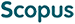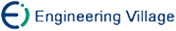郎璇聪, 李春生, 刘勇, 王梅. 基于稳定性分析的非凸在线点对学习的遗憾界[J]. 计算机研究与发展.
 引用本文: 郎璇聪, 李春生, 刘勇, 王梅. 基于稳定性分析的非凸在线点对学习的遗憾界[J]. 计算机研究与发展.Lang Xuancong, Li Chunsheng, Liu Yong, Wang Mei. Regret Bounds for Online Pairwise Learning with Non-Convex Losses Using Stability[J]. Journal of Computer Research and Development.
 Citation: Lang Xuancong, Li Chunsheng, Liu Yong, Wang Mei. Regret Bounds for Online Pairwise Learning with Non-Convex Losses Using Stability[J]. Journal of Computer Research and Development.## Regret Bounds for Online Pairwise Learning with Non-Convex Losses Using Stability

• 摘要: 点对学习是指损失函数依赖于2个实例的学习任务. 遗憾界对点对学习的泛化分析尤为重要. 现有的在线点对学习分析只提供了凸损失函数下的遗憾界. 为了弥补非凸损失函数下在线点对学习理论研究的空白，提出了基于稳定性分析的非凸在线点对学习的遗憾界. 首先提出了一个广义的在线点对学习框架，并给出了具有非凸损失函数的在线点对学习的稳定性分析；然后，根据稳定性和遗憾之间的关系，对非凸损失函数下的遗憾界进行研究；最后证明了当学习者能够获得离线神谕（oracle）时，具有非凸损失函数的广义在线点对学习框架实现了最佳的遗憾界O(T^ - 1/2).

Abstract: Pairwise learning refers to a learning task which involves a loss function depending on pairs of instances. Recently, there is a growing interest in studying pairwise learning since it includes many important machine learning tasks as specific examples, e.g., metric learning, AUC maximization and ranking. Regret bounds are particularly important for generalization analysis of online pairwise learning. The existing online pairwise learning analysis provided regret bounds only with convex loss functions. To fill the gap in the theoretical study of online pairwise learning with non-convex loss functions, we present a systematic study on the generalization analysis for online pairwise learning and propose regret bounds for non-convex online pairwise learning in this paper. We consider online learning in an adversarial, non-convex setting under the assumption that the learner has an access to an offline optimization oracle and the learner’s prediction with expert advice. We first propose a general online pairwise learning framework and establish the stability of online pairwise learning with non-convex loss functions. Then, the regret bounds can be derived naturally from stability. Finally, we show that the general online pairwise learning framework with non-convex loss functions achieves optimal regret of O(T^ - 1/2) when the learner has access to an offline optimization oracle./下载:  全尺寸图片 幻灯片
• 分享
• 用微信扫码二维码

分享至好友和朋友圈